## Table of ContentOpen Access

ARTICLE

# Numerical Assessment of Nanofluid Natural Convection Using Local RBF Method Coupled with an Artificial Compressibility Model

Muneerah Al Nuwairan1,*, Elmiloud Chaabelasri2

1 Department of Mathematics and Statistics, College of Science, King Faisal University, Al-Ahsa, 31982, Saudi Arabia
2 PTPME Laboratoy, Faculty of Sciences, Mohamed First University, Oujda, 60000, Morocco

* Corresponding Author: Muneerah Al Nuwairan. Email:Computer Modeling in Engineering & Sciences 2023, 135(1), 133-154. https://doi.org/10.32604/cmes.2022.022649

## Abstract

In this paper, natural heat convection inside square and equilateral triangular cavities was studied using a meshless method based on collocation local radial basis function (RBF). The nanofluids used were Cu-water or -water mixture with nanoparticle volume fractions range of . A system of continuity, momentum, and energy partial differential equations was used in modeling the flow and temperature behavior of the fluids. Partial derivatives in the governing equations were approximated using the RBF method. The artificial compressibility model was implemented to overcome the pressure velocity coupling problem that occurs in such equations. The main goal of this work was to present a simple and efficient method to deal with complex geometries for a variety of problem conditions. To assess the accuracy of the proposed method, several test cases of natural convection in square and triangular cavities were selected. For Rayleigh numbers ranging from to , a validation test of natural convection of Cu-water in a square cavity was used. The numerical investigation was then extended to Rayleigh number , as well as -water nanofluid with a volume fraction range of . In a second investigation, the same nanofluids were used in a triangular cavity with varying volume fractions to test the proposed meshless approach on non-rectangular geometries. The numerical results appear to be in agreement with those from earlier investigations. Furthermore, the suggested meshless method was found to be stable and accurate, demonstrating that it may be a viable alternative for solving natural heat transfer equations of nanofluids in enclosures with irregular geometries.

## Graphical Abstract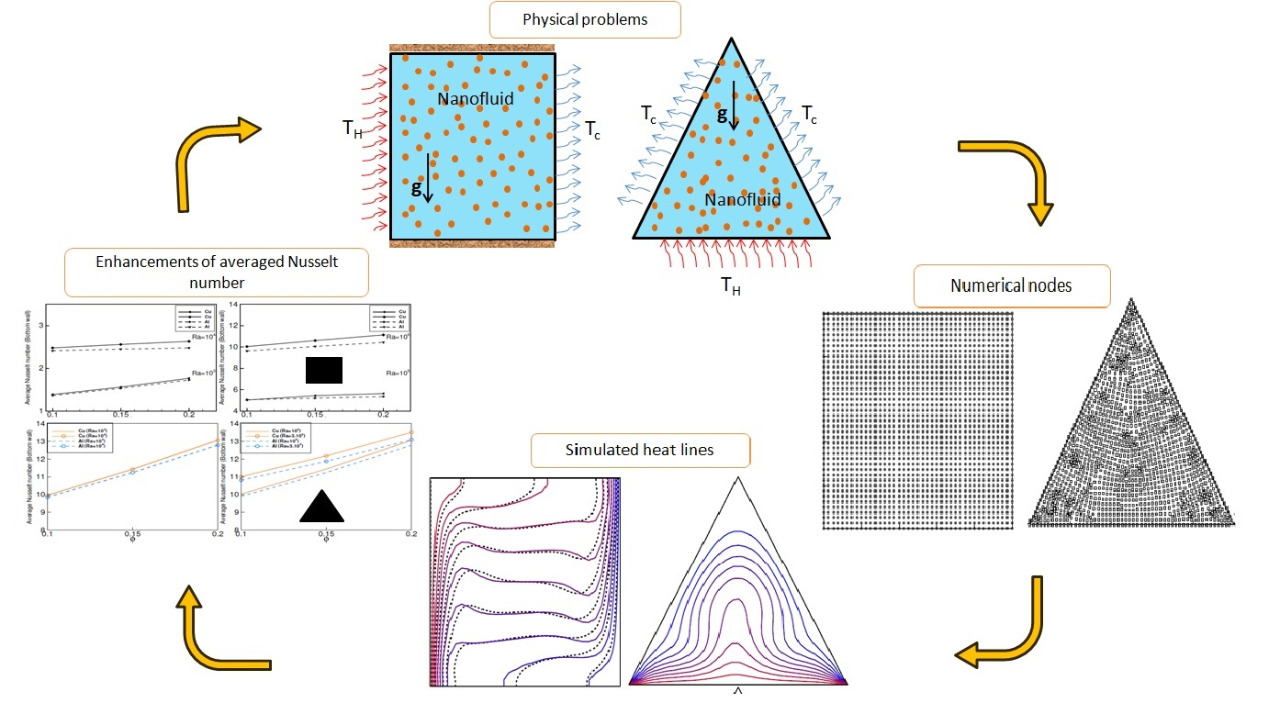## Keywords

Abbreviations

 N Number of nodes Cp Specific heat, J/kg/K g Acceleration due to gravity, m/s2 k Thermal conductivity, W/mK Nu Nusselt number Pr Prandtl number Ra Rayleigh number p Pressure, N/m2 P Dimensionless pressure T Temperature, K θ Dimensionless temperature u, v Dimensional velocity components in x and y, m/s U, V Dimensionless velocity components in X and Y x, y Dimensional Cartesian coordinates m X, Y Dimensionless Cartesian coordinates Dx, Dy First-order differentiation matrix Dxx, Dyy Second-order differentiation matrix Greek Symbols α thermal diffusivity, m2/s β thermal expansion coefficient, 1/K μ effective viscosity, m2/s ρ density, kg/m3 γ artificial compressibility parameter ε shape parameter ξ pseudo time Subscripts f base fluid nf nanofluid p solid particles c cold h hot

1  Introduction

In heat transfer, the heat convection occurs when heat is transferred from one part of the fluid to another. Natural convection has become an active research topic due to its lower cost and wide applications in engineering. Several models were developed for calculating the efficiency of thermal conductivity in solid and fluid systems . In , Choi showed that adding some nano metal particles to the base fluid increases its conductivity. Such mixtures, known as nanofluids, have many applications in science and engineering . These applications include fuel cells, hybrid-powered engines, chillers, and heat exchangers. The usefulness of a nanofluid for heat transfer applications can be verified by modeling the convective transfer in the nanofluid.

Several numerical methods, such as finite elements, finite volumes, and finite differences have been used to solve the thermal convection equations and simulate convective heat flow in various geometries. These methods are mesh based methods and are widely used. Several researchers have used these methods to model natural convection in nanofluids. Oztop et al.  used the method of finite volumes to investigate thermal transfer in a square cavity filled with a nanofluid. The investigation examined the effect of the size of the nanoparticles and the type of nanofluid. Mahmoodi et al.  used the same method with the SIMPLER algorithm to investigate heat transfer in a square cavity filled with nanofluid and having adiabatic square bodies at its center. These two studies found that increasing the volume fraction of the nanofluid increases heat transfer and that the type of nanoparticle is a key factor in heat transfer improvement. Jasim et al.  studied the influence of an inner adiabatic rotating cylinder on mixed convection of hybrid nanofluid in more complex geometries. Their research gave important insights for rotary heat exchanger designers. The set of conservation equations and their associated boundary conditions were discretized using the finite volume technique.

Finite elements have also been widely used. For example, Bhowmick et al.  used the Galerkin Finite Element Method to discretize the governing equations of natural convection heat transfer and entropy generation for a square enclosure, containing a heated circular or square cylinder subjected to non-uniform temperature distributions on the left vertical and bottom walls. The finite element method was also used by Islam et al.  to analyze temperature transfer within a prismatic cavity filled with Cu-water nanofluid under two different temperature boundary conditions.

It is worth noting that, over the last two decades, the lattice Boltzmann method has seen significant advances and is now commonly used in heat transfer problems, particularly for nanofluids. In Izadi et al. , natural convection of multi-wall carbon nanotubes-Iron Oxide nanoparticles/water hybrid nanofluid inside a -shaped enclosure was investigated. The effects of different parameters such as the nanoparticles volume fraction, the cavity obstruction ratio, the heat source aspect ratio, the heat source position, and the Rayleigh number on the hydrodynamic and thermal characteristics were studied using an in-house code based on the lattice Boltzmann method. Naseri Nia et al.  analyzed, using the lattice Boltzmann method, the effect of baffle configuration on natural convection inside an L-shaped enclosure filled with Cu-water nanofluid, for different parameter ranges of Rayleigh number and nanoparticle volume. Their main finding was that at high Rayleigh numbers, only the longer baffle improves convection regardless of its positioning, while increasing the volume fraction of nanoparticles always improves the thermal performance. To reduce the needed computations and to deal with more complex geometries, the meshless methods offer very good alternatives. These methods do not require a mesh, using instead a set of nodes in the space of the study domain.

Recently, Petrov-Galerkin local meshless method was used to study natural convection in a porous medium , Wijayanta et al.  analyzed a local radial basis function method to obtain numerical solutions of the conjugate natural convection heat transfer problem for square cavity. Zhang et al.  tested the robustness and accuracy of the variational multiscale element free Galerkin method, another meshless method, on several cases of natural convection, including a semicircular cavity, a triangular cavity with a flat wall, and a zigzag shape. The results obtained in these studies clearly showed that meshless methods are stable, accurate, and efficient. In addition to these classic methods, artificial neural networks (ANN) were used in studying convention heat transfer. An ANN based method was developed by Rostami et al.  to predict the thermal conductivity of multiwall carbon nanotubes-water and Cu-water nanofluids. Using experimental data, their algorithm selects ANNs with optimal performance on the prediction problem at hand. This study found that ANNs have excellent predictive ability and can make important contributions to the determination of the most desirable performance.

Other lines of investigation relevant to our study were explored. Kavusi et al.  studied the effect of different nanofluids, prepared using alumina, copper oxide and silver nanoparticles, at different concentrations and particle diameters on the performance of heat pipe, a heat exchange device with high efficiency and performance in heat transfer. Their study found that the use of a nanofluid in place of water resulted in an increase in thermal efficiency and a reduction in heat at the heat pipe wall. Rahmati et al.  simulated heat transfer in a microtube. The cooling fluid used was a combination of 1% and 1.5% volume fractions of CuO nanoparticles in a non-Newtonian pseudo-plastic fluid. Several challenges were identified in this work, namely the effect of slip velocity and the choice of the power law model for dynamic viscosity estimation. This study found that increasing the volume fraction of solid nanoparticles and the slip velocity coefficient resulted in an increase in heat transfer.

The present work aims to develop an accurate method using local radial basis function combined with the artificial compressibility model to solve the natural convective heat transfer problems in complex geometries. These methods have rarely been used in the case of nanofluids. A square cavity and an equilateral triangular cavity filled with a Cu-water or Al2O3-water based nanofluid with different volume fractions were studied. The use of a combination of the local radial basis function and the artificial compressibility method to solve the natural convection problems in a nanofluid is the main contribution of this work. The numerical algorithm uses the explicit two-stage Range-Kutta scheme for the pseudo-temporal discretization. We demonstrate that the radial basis function method allows for easy treatment of non-rectangular geometries. Indeed, the generation of collocation nodes in the computational domain and the treatment of boundary conditions do not require additional effort. In addition, we demonstrate that approximating the derivatives can be performed accurately using local radial basis functions.

This paper is structured as follows: The problem and the mathematical implementation of the governing equations are described in Section 2. The numerical formulas of the meshless approach are detailed in Section 3. In Section 4, the numerical method is applied and the numerical results are presented, along with a discussion of the accuracy and efficiency. Section 5 summarizes the results, adding some remarks and conclusions.

2  Problems Definition and Governing Equations

2.1 Problem Definition

To demonstrate the simplicity and efficiency of the proposed method to deal with complex geometries, we choose two domains. The proposed configurations are illustrated in Fig. 1, they consist of:

•   A two-dimensional square cavity. The left and right walls of the square cavity are the hot wall (Th) and the cold wall (Tc), respectively. The upper and lower horizontal walls of the cavity are kept adiabatic.

•   An equilateral triangular cavity where the bottom wall is the hot wall while the other two upper walls are kept adiabatic.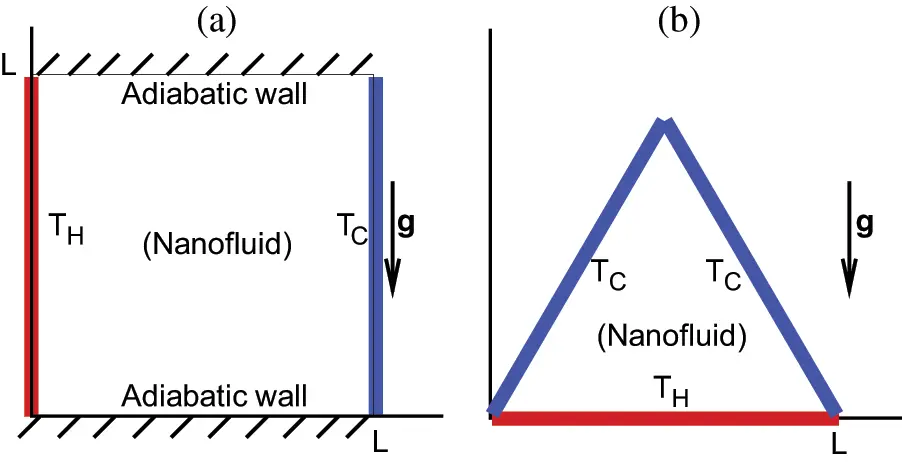Figure 1: Schematic geometry of physical problems: (a) Differentially heated square cavity and (b) Uniformly heated triangular cavity from bottom

The cavities considered are filled with a nanofluid composed of a nanoparticle-water mixture. The nanofluid is considered Newtonian, and the flow is laminar. The thermo-physical properties of the base fluid and the nanoparticle are taken as  and listed in Table 1.They are assumed constant except for the density which is given by the Boussinesq approximation.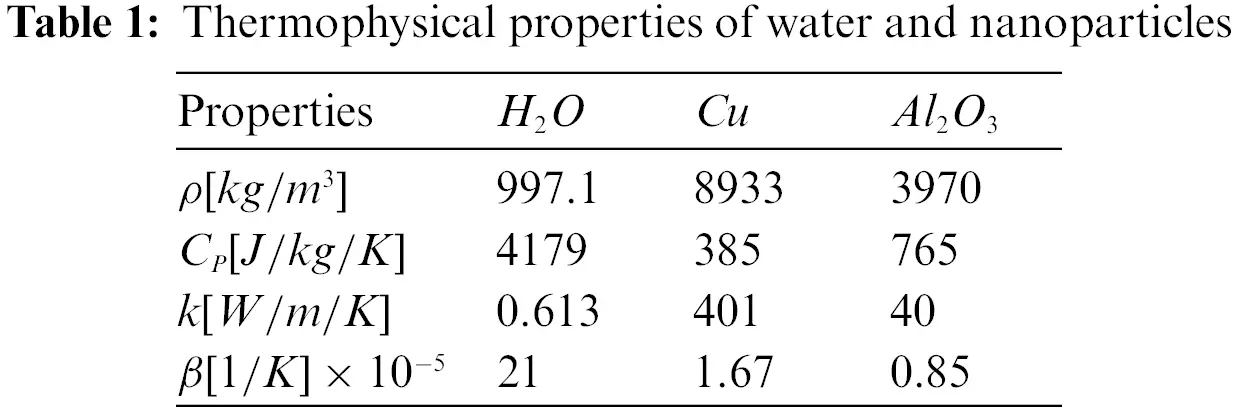2.2 Governing Equations

The equations of the continuity, momentum, and energy for buoyancy-driven laminar fluid flow and heat transfer of the nanofluid inside a cavity are

ux+vy=0(1)

ut+uux+vuy=1ρnfpx+μnfρnf(2ux2+2uy2)(2)

vt+uvx+vvy=1ρnfpy+μnfρnf(2vx2+2vy2)+g(ρβ)nfρnf(TTc)(3)

Tt+uTx+vTy=αnfρnf(2Tx2+2Ty2)(4)

where u and v are the components of the velocity in the x and y directions, T is the temperature, p is the pressure, the meaning of the other variables are found in the abbreviations at the end of the paper. Using the dimensionless variables:

X=xL,Y=yL,ξ=tαfL2,U=uLαf,V=vLαf,P=pL2ρnfαf2,θ=TTcThTc(5)

L is the characteristic length as well as the length of the cavity edge. For this work, we set it to 1. The above equations can be written in the dimensionless form:

UX+VY=0(6)

Uξ+UUX+VUY=ρfρnfPX+μnfαfρnf(2UX2+2UY2)(7)

Vξ+UVX+VVY=ρfρnfPY+μnfαfρnf(2VX2+2VY2)+(ρβ)nfβfρnfRaPrθ(8)

θξ+UθX+VθY=αnfαf(2θX2+2θY2)(9)

where Ra=gβf(ThTc)L3νfαf is the Rayleigh number, Pr=νfαf is the Prandtl number, ρnf is the density of nanoparticles, and μnf is the effective viscosity of the nanofluid. In this work, the dynamic viscosity of water is set μf=1.05103Pas, which gives a Prandtl number Pr=7.15.

For the cases studied, we have used three types of walls; hot, cold or adiabatic wall. The corresponding boundary conditions are

•   U(X,Y)=V(X,Y)=0 for all walls.

•   θ(X,Y)=0 for cold walls.

•   θ(X,Y)=1 for hot walls.

For calculating the local and average Nusselt numbers, the following formulas were used, respectively

Nuξ=knfkfθξ|ξ=0,Nuavg=LNudξ(10)

In this work, the values of the Nusselt number were calculated on horizontal or vertical walls, where for a horizontal wall ξ=y and for a vertical wall ξ=x.

2.3 Nanofluid Thermophysical Properties

The thermophysical parameters of the base fluid, such as density, viscosity, and heat conductivity, are affected by the addition of nanoparticles. In this study, the models that describe the thermophysical properties were obtained from the literature and are as follows:

The effective density of the nanofluid is given as

ρnf=(1ϕ)ρf+ϕρp(11)

where ϕ is the volume fraction of the nanoparticles, ρf is the density of base fluid and ρp is the density of nanoparticles. Thermal diffusivity of the nanofluid is given by

αnf=knf(ρCP)nf(12)

where knf is the effective thermal conductivity. Following Maxwell’s model  for spherical particles, we used the following expression:

knfkf=kp+2kf2ϕ(kfkp)kp+2kf+2ϕ(kfkp)(13)

Here kf is the thermal conductivity of the base fluid, and kp the thermal conductivity of dispersed particles. The heat capacity of the nanofluid introduced in Eq. (12) is given by

(ρCP)nf=(1ϕ)(ρCP)f+ϕ(ρCP)p(14)

The thermal expansion coefficient of the nanofluid is given by

(ρβ)nf=(1ϕ)(ρβ)f+ϕ(ρβ)p(15)

Finally, the effective viscosity of the nanofluid, based on Brinkman model , is given by:

μnf=μf(1ϕ)2.5(16)

where μf is the viscosity of the base fluid.

3  Numerical Method

3.1 Radial Basis Function (RBF) Method

We employ the radial basis functions method, which is a meshless method originally proposed by Kansa , for the numerical solution of natural convection equations. In the following, we outline the main principles of this method.

Radial basis function interpolation approximates the solution function by an expansion. According to the Kansa method, the solution is approximated on a set of N collocation nodes by a linear combination of local radial basis function as follows :

F(x,t)=jsi,nλF,jϕ(xxj,ε)(17)

where λF,j are the unknown coefficients to be calculated, ϕ(x) is a radial basis function, xxj is the distance between the nodes x and xj, and si,n is a stencil that contains the index i and indices of the n nearest neighboring of the node xi. The stencil consists of the indices of node points falling within three times the distance to the nearest node. Thus, the number of nodes indexed by the stencil is variable, as it depends on the distribution of nodes in the computational domain.

Many radial basis functions were proposed In the literature. In the current study, we use the infinitely smooth multiquadric radial basis function defined as ϕ(xxj)=1εxxj2, where ε0 is a shape parameter that plays a substantial role in the resulting accuracy. Generally, the determination of the range of shape value can be obtained by using numerical tests. In this study, we, in addition, use the procedure proposed by Sarra in . Therefore, an optimal shape parameter has been identified. In all simulations, ε=ε0ndmin, in which dmin is the minimum distance between two nodes and ε0=102 for square cavity and ε0=0.15 for triangle cavity.

The numerical solution of an equation involving partial derivatives can be approximated by using linear approximation of the partial derivatives at the x nodes, based on local radial basis functions as follows:

LF(x,t)=jsi,nλF,jLϕ(xxj,ε)(18)

This can be rewritten in a more concise form as

LF[i]=ΦL[i]ΛF[i](19)

where ΛF[i]=[λF,1,λF,2,λF,n]T is an n×1 vector of unknown coefficients to be calculated and ΦL is an n×n matrix containing linear differentiation of radial basis function Lϕ(xixj,ε). Using Eq. (17), the coefficients ΛF can be expressed in terms of the function F as follows:

ΛF[i]=(Φ[i])1F[i](20)

where (Φ[i])1 is the inverse of the matrix Φ[i]. By removing the dependence on the expansion coefficients, the equation in (19) becomes:

LF[i]=ΦL[i](Φ[i])1F[i](21)

Note that in the governing equations the first and second derivatives are calculated for each node by using several matrix operations on n×n matrices. This slowdown the calculation process. In order to overcome the problem of successive inversion of many n×n matrices, Yao et al.  reformulated the local interpolation and differentiation matrices in terms of a global sparse matrix by appending zeros to the proper entries. This reformulation was also used by  for the space-time derivatives. The entries of the global differentiation matrix DL are then defined as follows:

DL(i,j)={ΦL[i](Φ[i])1ifjsi,n0otherwise(22)

Using this approach, the first and second spatial derivatives of a function F(x,t) can be expressed as follows:

F(x,t)x=DxF(x,t);F(x,t)y=DyF(x,t);(23)

2F(x,t)x2=DxxF(x,t)andF(x,t)y2=DyyF(x,t)(24)

where Dx,Dy,Dxx and Dyy are first and second derivative matrices with respect to x and y.

3.2 Numerical Procedure

In attempting a numerical solution of the Eqs. (6)(9), one is faces with several difficulties. The momentum equations contain a pressure gradient, moreover, these equations can not be solved if the pressure term is not specified. The method of artificial compressibility proposed by Chorin  is adopted in this work for the treatment of the pressure-velocity coupling. This method adds a pseudo-temporal derivative to the continuity equation to couple pressure with velocity, as follows:

Pξ+γ(UX+VY)=0(25)

Here γ is an artificial compressibility parameter, a free but important parameter that affects the convergence of the solution. In this study, to obtain the convergence of the solution, a value of 105 was employed.

For simplicity, the Eqs. (25), (7)(9) are rewritten in a compact form as follows:

Wξ=L(W)(26)

where,

W=[P(t),U(t),V(t),θ(t)]T;P(t)=[P1(t),P2(t),,PN(t)]T;U(t)=[U1(t),U2(t),,UN(t)]T;V(t)=[V1(t),V2(t),,VN(t)]T;θ(t)=[θ1(t),θ2(t),,θN(t)]T

with the superscript T denoting the transpose and

L(W)=[γ(UX+VY)UUXVUYρfρnfPX+μnfαfρnf(2UX2+2UY2)UVXVVYρfρnfPY+μnfαfρnf(2VX2+2VY2)+(ρβ)nfβfρnfRaPrθUθXVθY+αnfαf(2θX2+2θY2)].(27)

On a set of N nodes, and using the differentiation matrix defined above, the right-hand side of the partial differential problem (25) can be approximated as

L(W)=[γ(DxU+DyV)UDxUVDyUρfρnfDxP+μnfαfρnf(DxxU+DyyU)UDxVVDyVρfρnfDYP+μnfαfρnf(DxxV+DyyV)+(ρβ)nfβfρnfRaPrθUDxθVDYθ+αnfαf(Dxxθ+Dyyθ)].(28)

The problem in Eq. (26) is integrated explicitly using the second Runge-Kutta method. Hence, we divide the pseudo-time into intervals [ξn,ξn+1], and denote Wn the value of the function W at time ξn. The recursive computation is given by

Wn+1=12[(Wn+W)+L(W)](29)

where

W=Wn+L(Wn)(30)

and L(Wn) is given in Eq. (26).

It should be noted that radial basis function approaches for time-dependent partial differential equations with diffusive terms can be stably advanced in time with a suitable choice of time step size . Although, the equations used in our study are time dependent, the aim of the study is to examine the steady-state solution. Thus, the method is used to reach the steady-state as the limit when the variations of variables approaches zero. The convergence criteria for steady state is

θn+1θnεerr(31)

and

Un+1Unεerr(32)

Here n and n+1 denote two adjacent time steps and εerr is taken equal to 105 in all cases.

3.3 Grid Independence and Validation Tests

Several preliminary tests were performed to evaluate the sensitivity of the results to the number of nodes. These tests were used to determine the optimal distribution of nodes that ensures good accuracy with reasonable computational cost, Three distributions are used for this investigation and are shown in Fig. 2. For these tests Cu-water nanofluid with volume fraction ϕ=0.2 is considered as the working fluid, and the Rayleigh number is taken as the constant 105. A horizontal cross section at y=0.5 of the horizontal velocity U was made and shown in Fig. 3. The same figure shows the variation of the local Nusselt number at the left wall. From these figures, it can be observed that the solutions become independent of the node distribution from 31×31. Therefore, to ensure good accuracy of the results, the distribution 41×41 was selected for the numerical investigations.Figure 2: Nodes distribution used for the study of grid independence for square cavity. 21 × 21 to the left, 31 × 31 at the middle and 41 × 41 to the right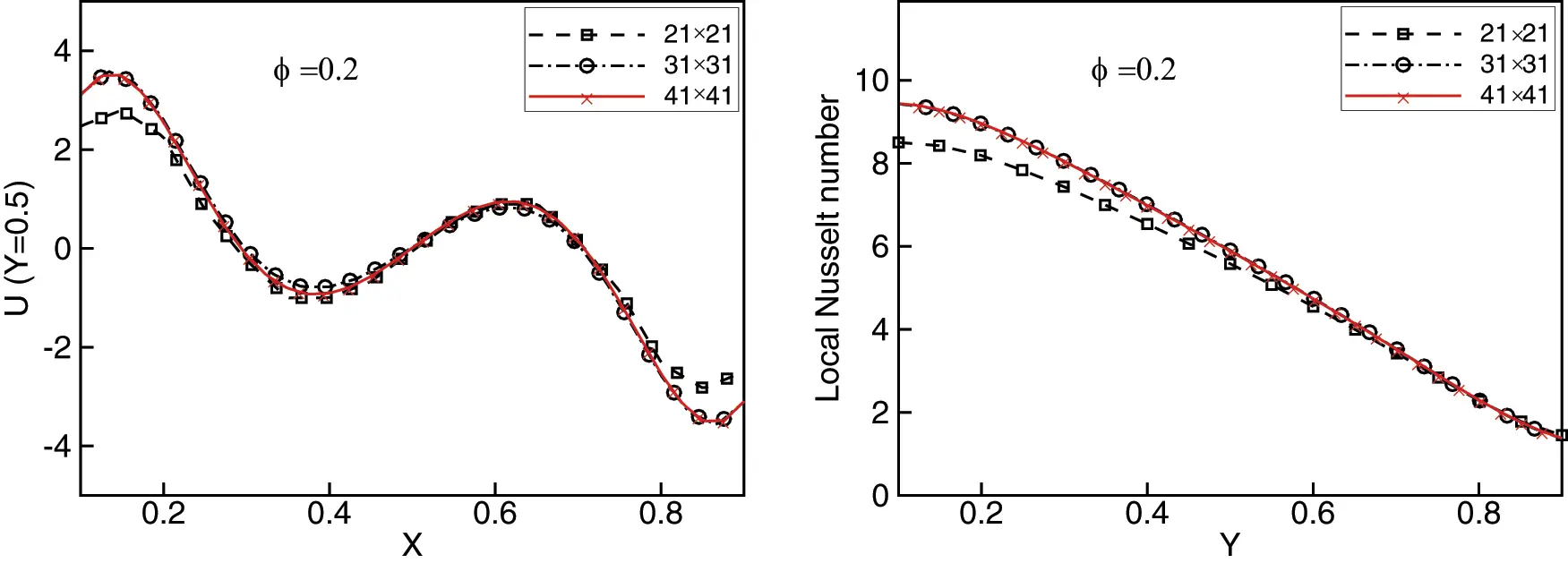Figure 3: Grid independence tests for variations of the U component of the velocity vs. X coordinate at Y = 0.5 (left) and local Nusselt number vs. Y coordinate at left wall (right)

The meshless method proposed in this work was then validated in the case of natural convection on two problems. The first was the natural convection in a cavity filled with pure water. In the second, we considered the Cu-water nanofluid with a volume fraction ϕ=0.2. The results are shown in Figs. 4 and 5. The results presented in Fig. 4 in terms of local Nusselt number at left wall for various Rayleigh number values, show that our code satisfactorily reproduces the results reported in . A comparison of the graphs in Fig. 5 of average Nusselt for different Rayleigh numbers shows that our results are in excellent agreement with those obtained in .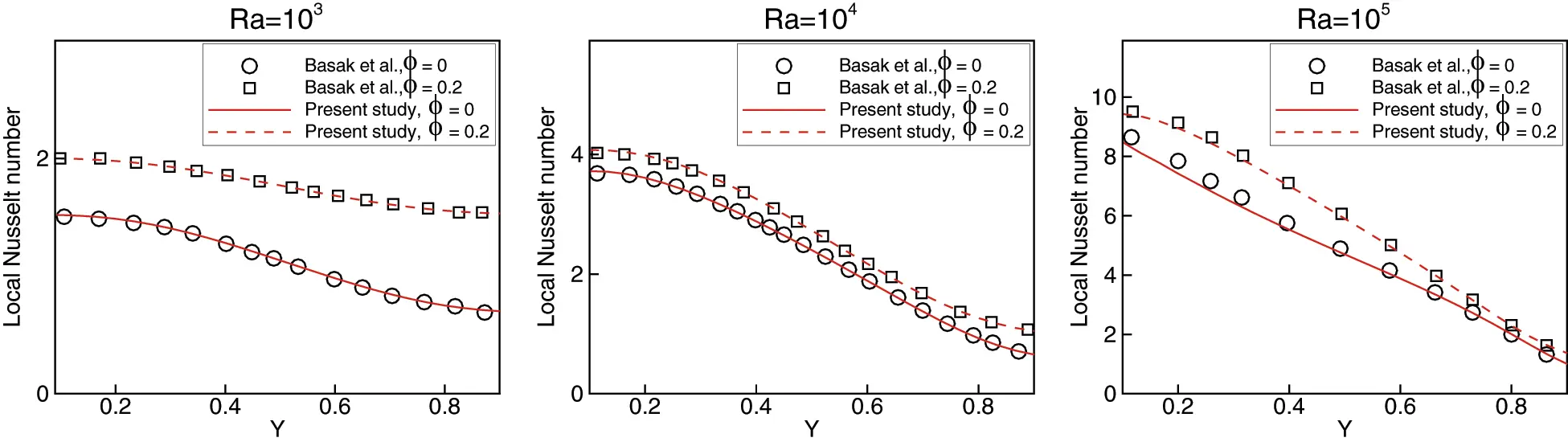Figure 4: Validation cases in Basak et al.  and present study. local Nusselt number vs. Y coordinate at left wall for Ra = 103, 104 and 105 and for Cu-water nanofluid with ϕ = 0.2 and pure water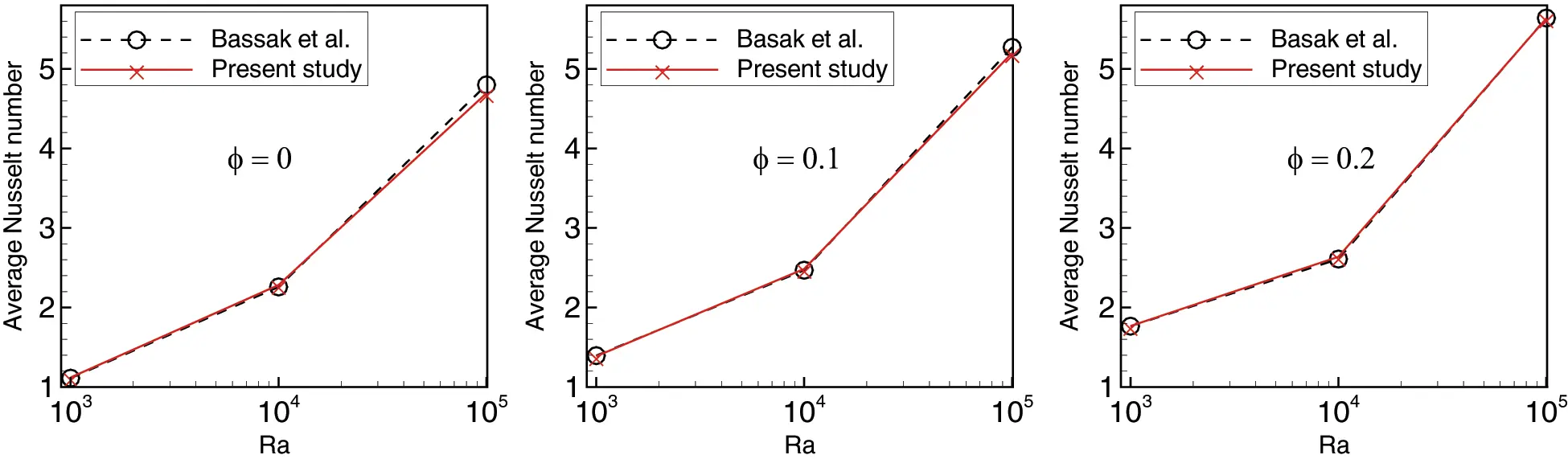Figure 5: Validation cases in Basak et al.  and present study. Average Nusselt number vs. Rayleigh for ϕ = 0 , ϕ = 0.1 and ϕ = 0.2 of Cu-water nanofluid

4  Results and Discussion

4.1 Differentially Heated Square Cavity

In the first investigation, we ran a simulation of the effects of differentially heated boundary conditions on natural convection in a square cavity filled with a nanofluid. We used the cavity previously for the validation of meshless method, but extend the range of the Raylieh number to 106 using nanofluids composed of different volume fractions ϕ=0.1, 0.15 and 0.20 using two types of nanoparticles, namely Cu and Al2O3. Their characteristics are listed in Table 1.

Figs. 6 and 7 show isotherms distributions for dilute Cu-water and Al2O3-water nanofluids. Isotherms are also shown for pure water for comparisons. Results are shown for all ranges of 103Ra106 and volume fractions ϕ=0.1, 0.15, and 0.20. Overall, the effect of adding nanoparticles is seen in all ranges of the Rayleigh number, as well as for the various proposed volume fractions. We also note that the convective heat flux for water is stronger near the center in all cases, particularly for high Rayleigh numbers and that the isotherms for water are more twisted at the center than those for the Cu-water or Al2O3-water nanofluid. Near the wall, the isotherms for Cu-water and Al2O3-water are different from those of water. These observations can be explained by the suppression of convection at low Rayleigh numbers. This also explains why the iso-lines are nearly vertical lines. In contrast, convection improves rapidly at the center of the cavity with increasing Rieylegh number reaching high ratios for Ra = 106. Finally, as Rayleigh number and volume fraction grow, the intensity of heat transfer increases, and the behavior of isotherms for Ra=105 and 106 are identical.Figure 6: Isotherms of differentially heated square cavity with a hot wall on the left, cold wall on the right, of pure water (dotted line) and a Cu-water nanofluid (continued), for volume fraction ϕ = 0.1, 0.15 and 0.2, and the numbers of Ra = 103, 104, 105 and 106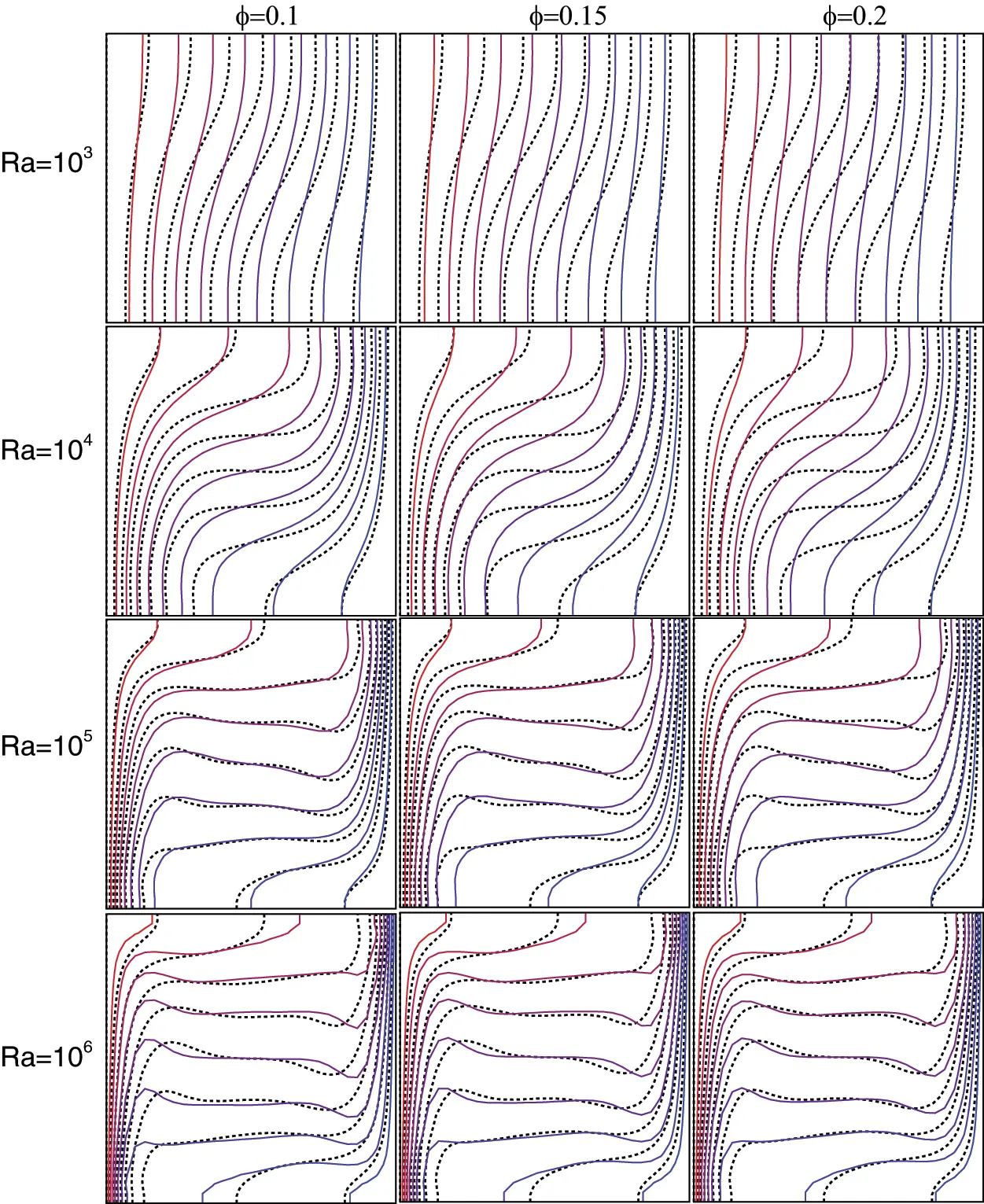Figure 7: Isotherms of differentially heated square cavity with a hot wall to the left, cold wall to the right, of pure water (dotted line) and a Al2O3-water nanofluid (continued), for volume fraction ϕ = 0.1, 0.15 and 0.2, and the numbers of Ra = 103, 104, 105 and 106

Figs. 8 and 9 illustrate the variations in the local Nusselt number along the heated wall for different values of the Rayleigh number and different volume fractions for the Cu-water and Al2O3-water nanofluid, respectively. In general, the Rayleigh number has a significant impact on the variation of the local Nusselt number, the heat transfer rate increases with rising Rayleigh number due to higher buoyancy forces. For Ra=103, the variation in local Nusselt number is small, but it grows for other values of Ra. The local Nusselt number has its highest values near the bottom of the wall, and it decreases gradually as it goes up the wall. This is mainly due to the decrease in the buoyancy of nanoparticles due to the gravitational force. This increases the conductivity of the nanofluid at the bottom of wall. It should be noted that increasing the volume fraction of nanoparticles in Cu or Al2O3 water, increases the conductivity of the nanofluid and consequently the increases the local Nusselt number. Thus, the natural heat convection is better enhanced with copper nanoparticles.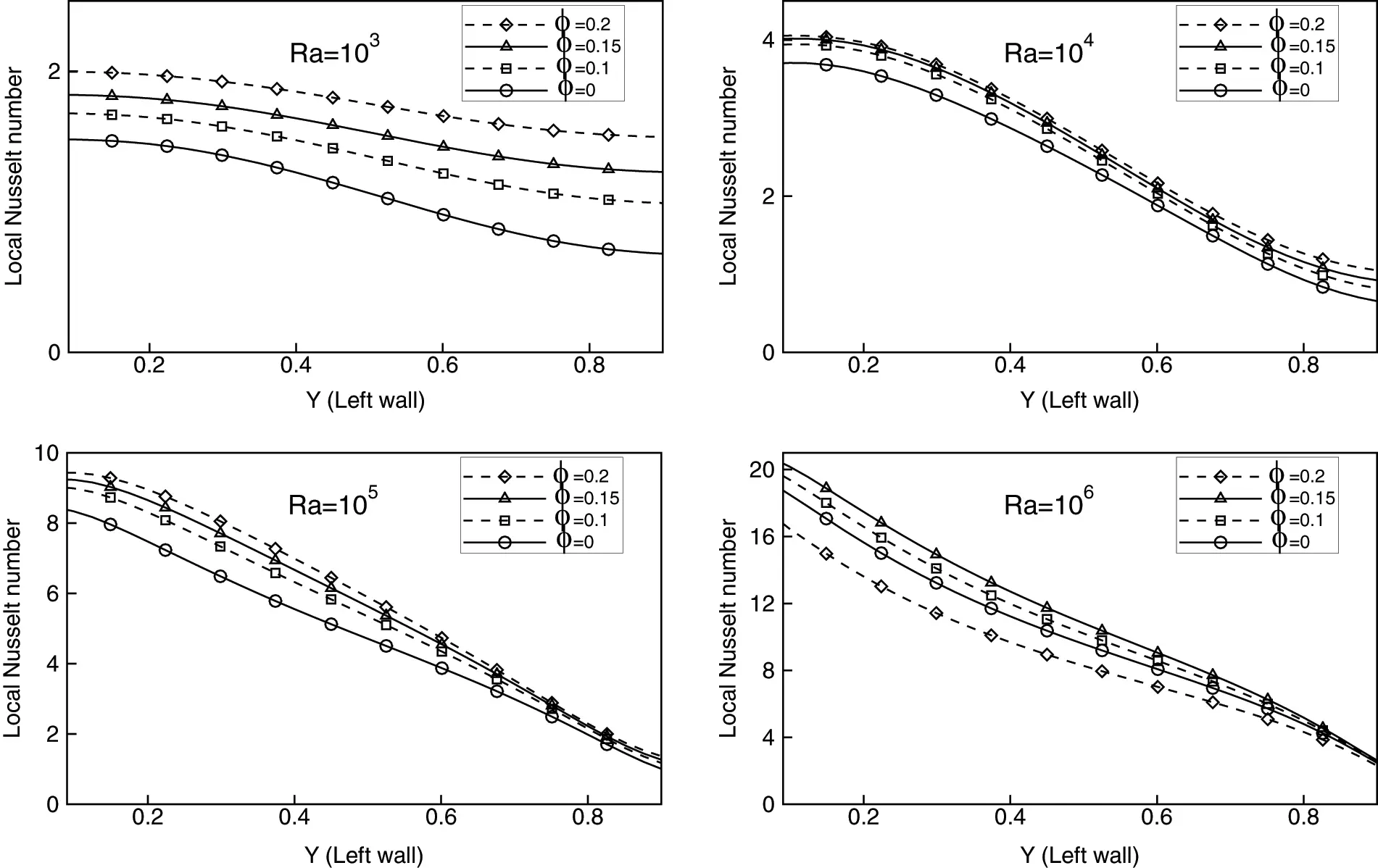Figure 8: Local Nusselt number at the left wall for Cu-water nanofluid with the volume fractions ϕ = 0, 0.1, 0.15 and 0.2 at Rayleigh numbers 103, 104, 105 and 106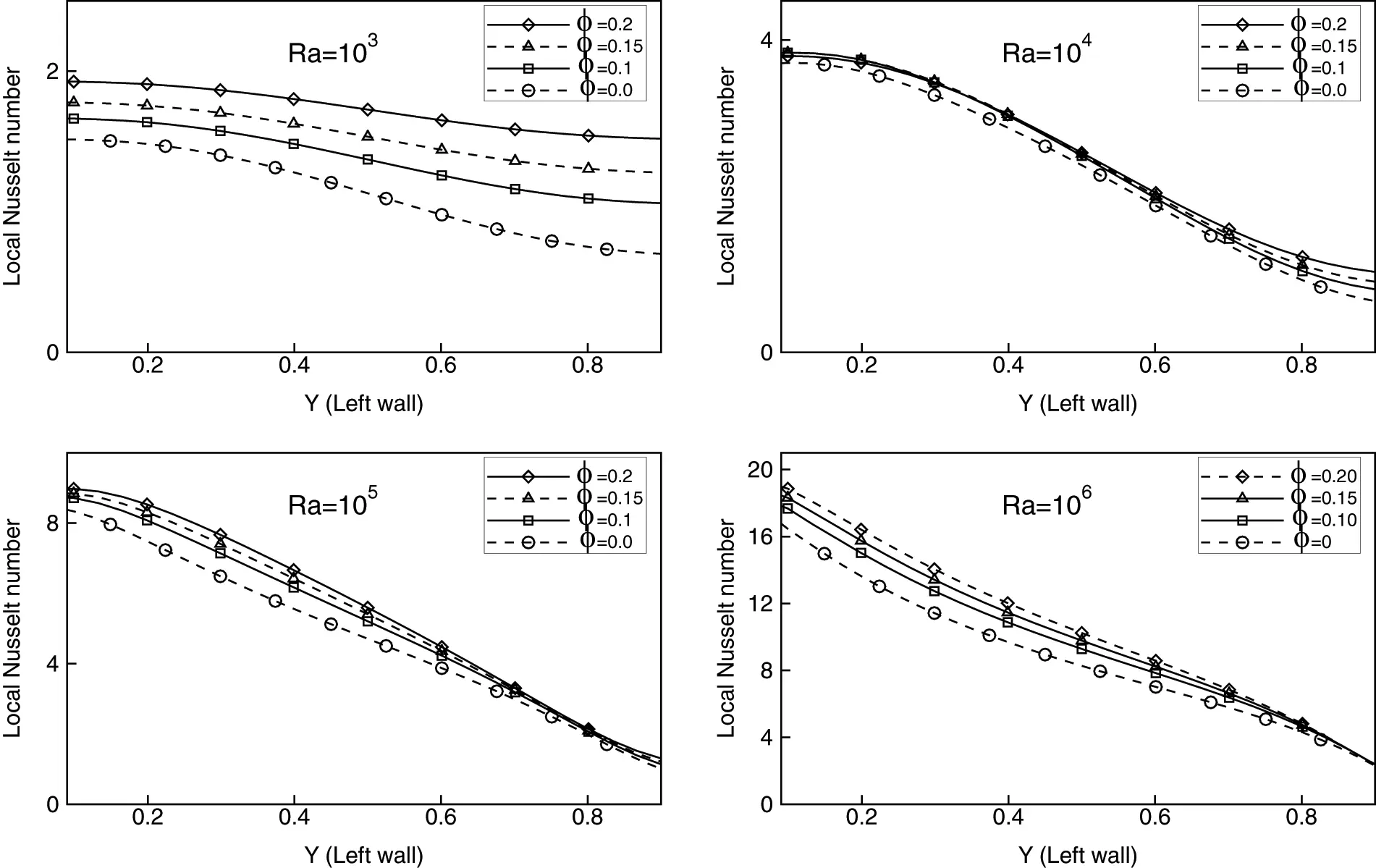Figure 9: Local Nusselt number at the left wall for Al2O3-water nanofluid with the volume fractions ϕ = 0, 0.1, 0.15 and 0.2 at Rayleigh numbers 103, 104, 105 and 106

Fig. 10 shows the variation of average Nusselt number against nanoparticle volume fraction for Ra=103, 104, 105 and 106. The average Nusselt number is sensitive to nanoparticle type and volume fraction, as shown in the Figures. It is worth noting that the Cu-water and Al2O3-water nanofluids followed similar trend. At low Rayleigh numbers, the effect of nanoparticles and the growth in their volume fractions is more relevant than at high Rayleigh numbers. As an example, for Cu-water nanofluid, the slope of the Rayleigh number against volume fraction is nearly at 20% for Ra=103 and 10% at Ra=106. The Al2O3-water exhibits the same behavior. This can be explained by the fact that at low Rayleigh numbers, conduction dominates heat transfer and viscous effects are less important. As a result, the inclusion of high thermal conductivity nanoparticles will improve conduction. The viscous effects, on the other hand, become more pronounced with large Rayleigh numbers. Finally, comparisons with previous results of averaged Nusselt number in Basak et al.  shows that our results are in good agreements with theirs. Therefore, the meshless method based on radial basis functions coupled with the artificial compressibility, described in this work, can be used with confidence for the simulation in such problems.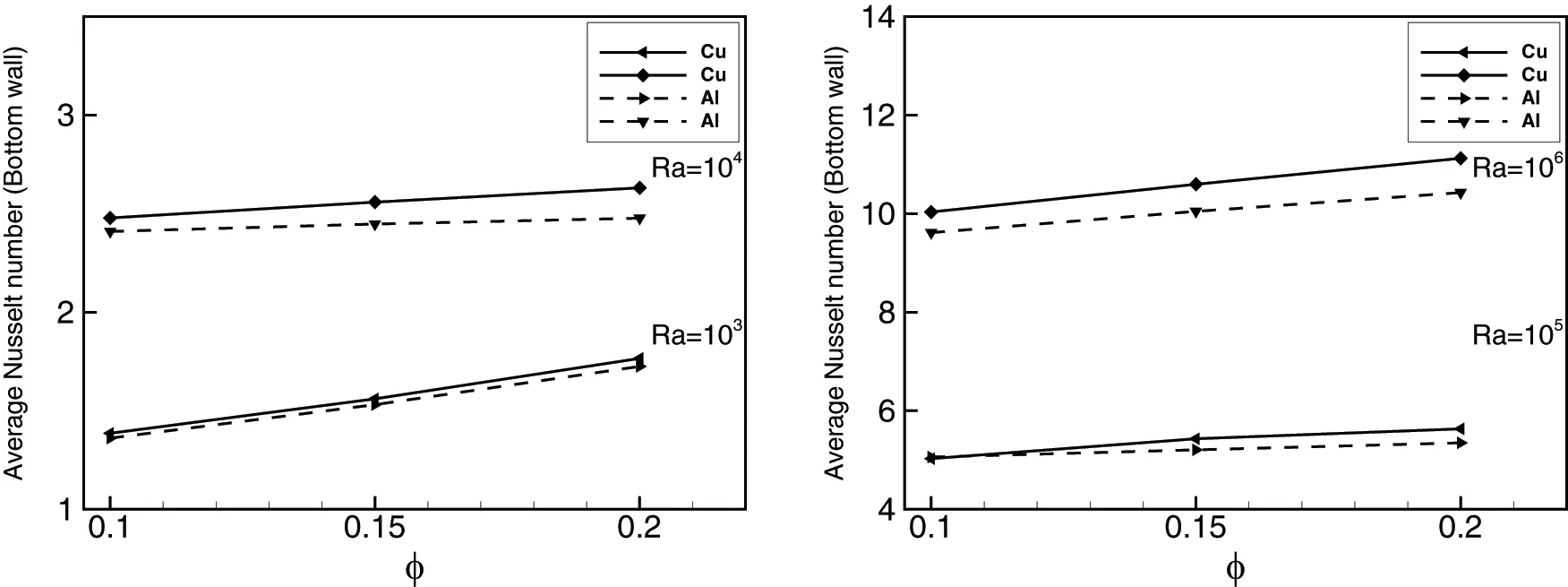Figure 10: Comparison and variation of average Nusselt number with volume fractions of Cu and Al2O3 nanoparticles at different Rayleigh number

4.2 Uniform Heated Triangular Cavity

In the second investigation, we looked at the natural convection in an equilateral triangular enclosure. The two nanofluids employed in the previous case were used. The two inclined walls of the cavity were kept cold, while the bottom wall is heated. Pranowo et al.  used a similar configuration filled with air to test the performance of their meshless method. The nodes distribution used for the numerical computation (Fig. 11) contains 1043 non-uniformly distributed nodes. Figs. 12 and 13 show the impact of Rayleigh number and volume fraction of nanoparticles on isotherms. As expected, for low Rayleigh numbers thermal conduction dominates. For the case of the triangular cavity this dominance occurs at Rayleigh numbers lower than 104. Thereafter, the behavior of the isotherms remains unchanged with the same pattern around the vertical plane at x=0.5. Indeed, the high viscosity of the nanofluid negatively affects the flow, leading to a slower convection and a decrease in heat transfer. As the Rayleigh number increases, so does the buoyancy force, resulting in an increase in natural convection, this results in the distortion towards the top of the isotherm.Figure 11: Node distribution used for numerical studies for triangular cavity, 1043 non-uniformly distributed nodes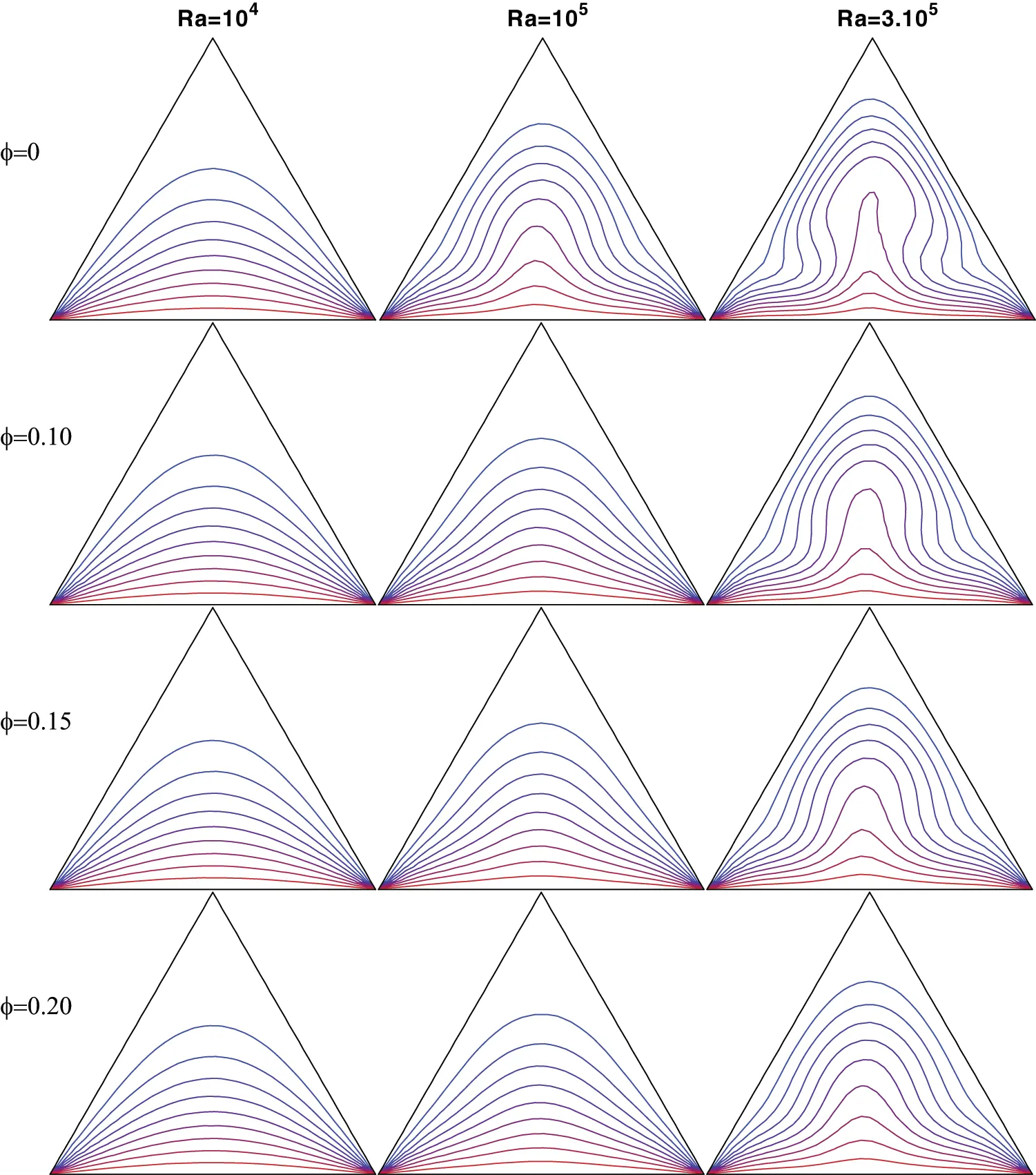Figure 12: Isotherms of uniformly heated triangular cavity with a hot wall on the bottom, cold lateral walls, of Cu-water nanofluid, for volume fraction ϕ = 0.1, 0.15 and 0.2, and Rayleigh numbers of Ra = 104, 105 and 3.105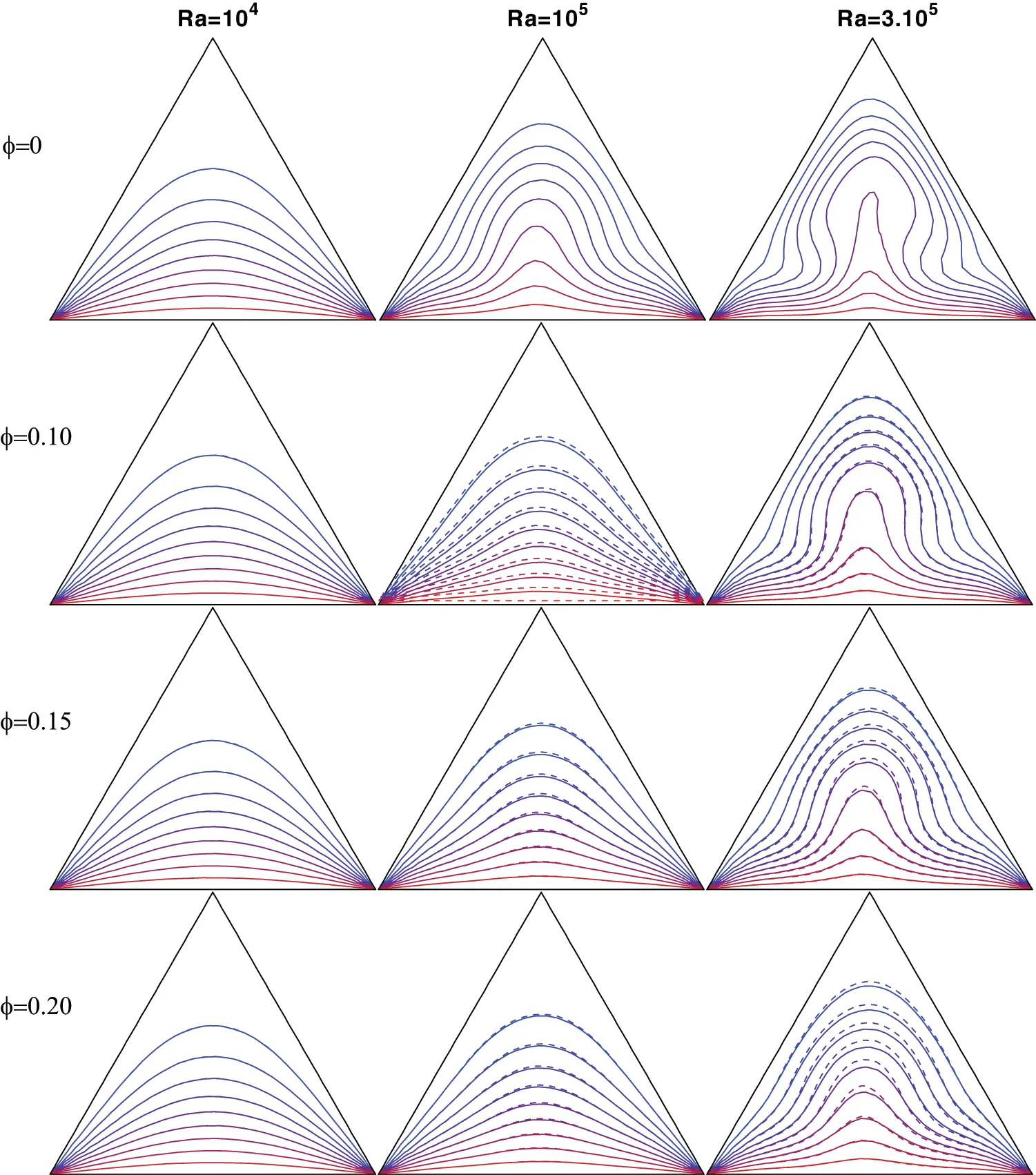Figure 13: Isotherms of uniformly heated triangular cavity with a hot wall on the bottom, cold lateral walls, of Al2O3-water nanofluid (continued) and Cu-water (doted line), for volume fraction ϕ = 0.1, 0.15 and 0.2, and Rayleigh numbers of Ra = 104, 105 and 3.105

Figs. 12 and 13, for Ra=106, indicate the dominance of convection. It should be noted that in spite of the high viscosity, the flow intensity is significantly improved for large Rayleigh values. Consequently, heat is clearly transferred from the hot bottom wall to the cold side walls. Furthermore, the heat transfer of the two nanofluids fits the same pattern seen in the case of the square cavity, with the heat transfer being better for the Cu-water nanofluid than Al2O3-water.

In order to quantify the heat exchange within the cavity filled with Cu-water and Al2O3-water, the effects of the volume fraction of both nanoparticles on the local Nusselt number along bottom wall are shown in Figs. 14 and 15 for Ra=104, 105 and 3.105. The smallest values of the local Nusselt number are observed in the middle of the heated part, where the temperature gradient is the smallest according to the isotherms shown in Figs. 12 and 13. It should be noted that the addition of nanoparticles increases the thermal conductivity of the nanofluid, and thus the heat transfer, which results in an increase in the local Nusselt number. Fig. 16 illustrates a comparison of the local Nusselt number for the two nanofluids. The Cu-water mixture remains a more conductive nanofluid, promoting heat transfer from the hot to cold walls.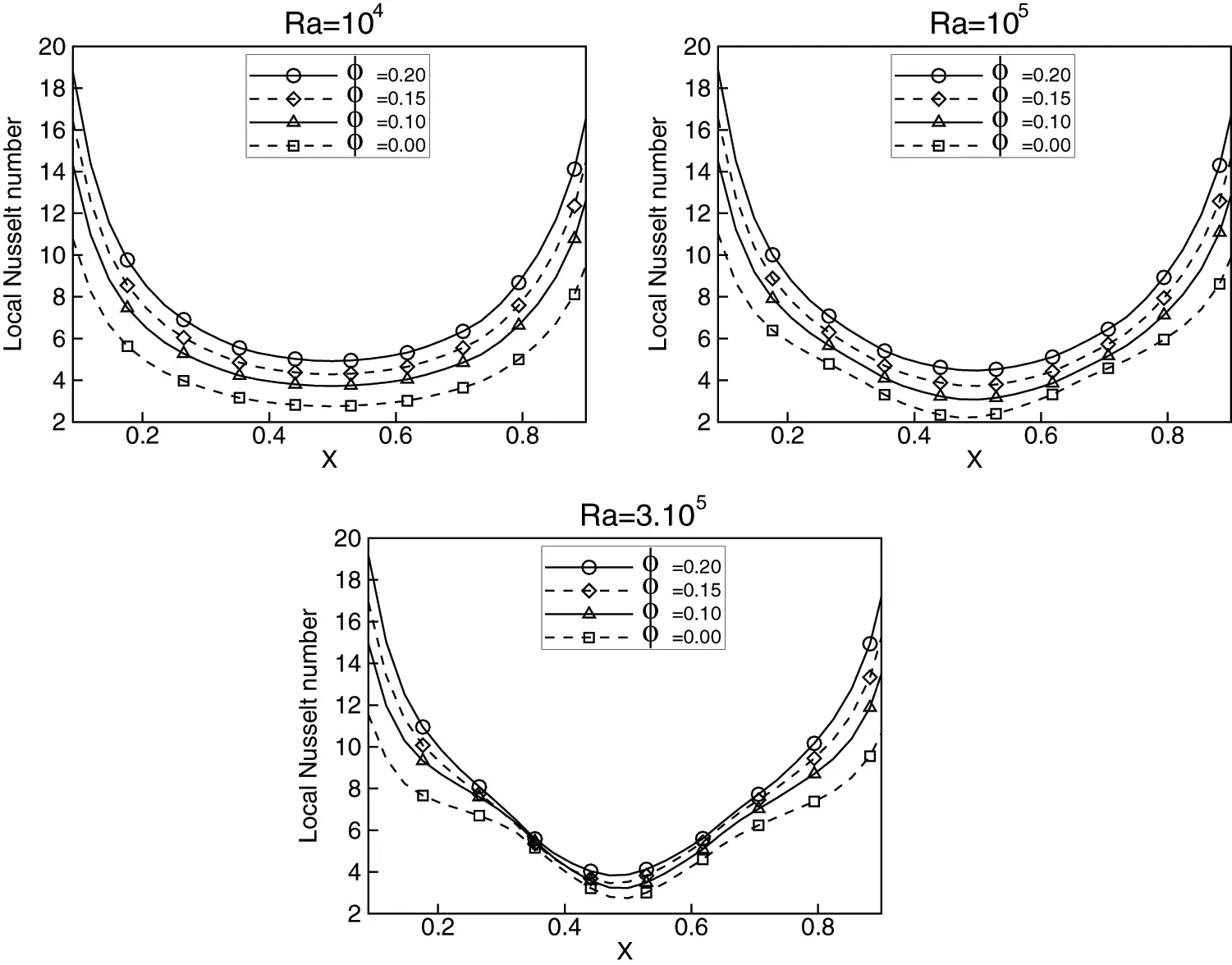Figure 14: Local Nusselt number at the bottom wall for Cu-water nanofluid with the volume fractions ϕ = 0, 0.1, 0.15 and 0.2 at Rayleigh numbers 104, 105 and 3.105Figure 15: Local Nusselt number at the bottom wall for Al2O3-water nanofluid with the volume fractions ϕ = 0, 0.1, 0.15 and 0.2 at Rayleigh numbers 104, 105 and 3.105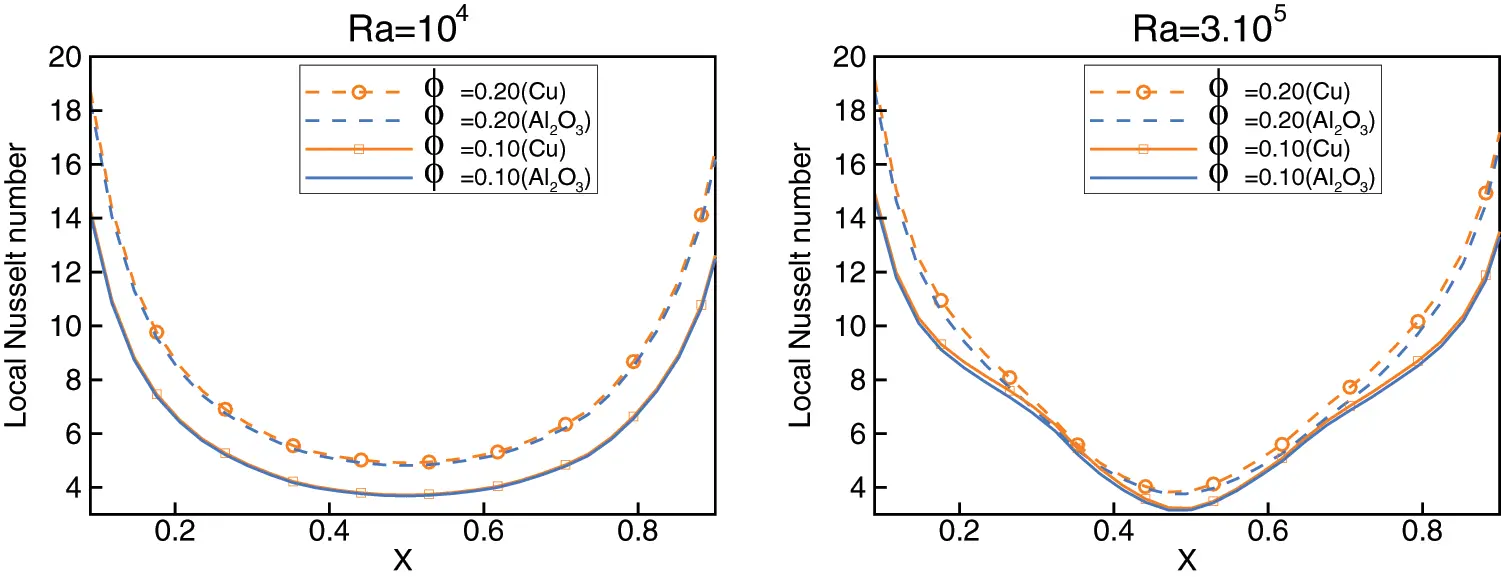Figure 16: Comparison of local Nusselt number at the bottom wall for Cu-water and Al2O3-water nanofluid with the volume fractions ϕ = 0.10 and 0.2 at Rayleigh numbers 104 and 3.105

Fig. 17 shows the variation of the average Nusselt number as a function of the volume fraction for various values of the Rayleigh number. As can be seen, increasing the volume fraction results in higher average Nusselt number, this is due to the increased conductivity achieved by adding nanoparticles. Furthermore, these results confirm that Cu-water nanofluid is a better heat transfer fluid than Al2O3-water nanofluid when used in a triangular cavity, and this appears only for high Rayleigh numbers.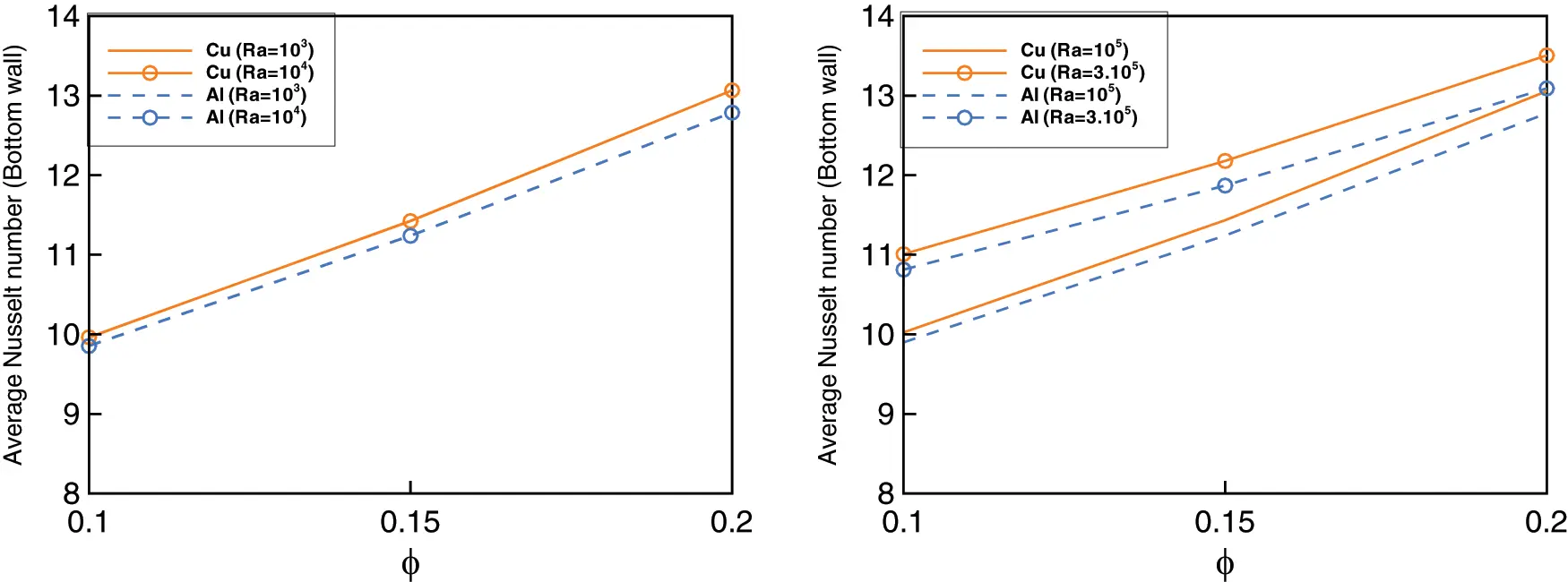Figure 17: Variation of average Nusselt number with volume fractions of Cu and Al2O3 nanoparticles at different Rayleigh number

5  Conclusions

A two-dimensional numerical investigation of natural convection in enclose cavities was carried out in this study. Two geometries were used, namely those of a square and a triangular cavity. The laminar heat transfer equations of nanofluid were solved using the radial basis function method coupled with an artificial compressibility, which is classified as a meshless method. Cu-water and Al2O3-water nanofluids with various volume fractions of nanoparticles at different Rayleigh numbers, starting at 103 and going up to 106, were studied. The following are the main findings of the study:

•   The implemented meshless method offers high flexibility in dealing with complex geometries due to the simplicity of the numerical evaluations of space derivative. This method provides a valuable and efficient way to analyze natural convection in nonrectangular geometries.

•   To overcome the pressure velocity coupling problem that occurs in equation systems like the ones examined, the artificial compressibility model was implemented. The quality of the results was found to be of the same accuracy order as the other classical methods.

•   Results clearly indicate that the addition of nanoparticles has produced a substantial enhancement of heat transfer as compared to that of the pure fluid. As mentioned above, convective transfer dominates conduction when Rayleigh numbers are increased. As a consequence, heat transfer from the hot walls to the cool walls is improved. This remains true for both of the cases investigated. Furthermore, we conclude that the heat transfer enhancement is not clear in low Rayleigh numbers or when the enclosures have geometric singularities, as was the case in our study, for the case of the triangular cavity.

Acknowledgement: The authors would like to acknowledge the financial support from King Faisal University, Saudi Arabia, Project No. AN000675.

Funding Statement: This work was supported through the Annual Funding Track by the Deanship of Scientific Research, Vice Presidency for Graduate Studies and Scientific Research, King Faisal University, Saudi Arabia [Project No. AN000675].

Conflicts of Interest: The authors declare that they have no conflicts of interest to report regarding the present study.

## References

1. Yu, W., & Choi, S. U. S. (2003). The role of interfacial layers in the enhanced thermal conductivity of nanofluids: A renovated Maxwell model. Journal of Nanopartical Research, 5, 167-171. [Google Scholar] [CrossRef]
2. Wasp, E. J., Kenny, J. P., Gandhi, R. L. (1979). Solid-liquid flow slurry pipeline transportation. Switzerland: Trans Tech Publications.
3. Souayeh, B., Ali Abro, K., Alfannakh, H., Al Nuwairan, M., & Yasin, A. (2022). Application of Fourier sine transform to carbon nanotubes suspended in ethylene glycol for the enhancement of heat transfer. Energies, 15, 1200. [Google Scholar] [CrossRef]
4. Hamilton, R. L., & Crosser, O. K. (1962). Thermal conductivity of heterogeneous two component systems. Industrial & Engineering Chemistry Fundamental, 1(3), 187-191. [Google Scholar] [CrossRef]
5. Maxwell-Garnett, J. C. (1904). Colours in metal glasses and in metallic films. Philosophical Transactions of the Royal Society of London. Series A, Containing Papers of a Mathematical or Physical Character, 203, 385-420. [Google Scholar] [CrossRef]
6. Al Nuwairan, M., & Souayeh, B. (2021). Augmentation of heat transfer in a circular channel with inline and staggered baffles. Energies, 14(24), 8593. [Google Scholar] [CrossRef]
7. Choi, S. U. S. (1995). Enhancing thermal conductivity of fluids with nanoparticles. In: Siginer, D. A., Wang, H. P. (Eds.), Developments and applications of non-newtonian flows, vol. 66, pp. 99--103. New York: The American Society of Mechanical Engineers.
8. Das, S., Choi, S., Wenhua, Y., Pradeep, T. (2007). Nonofluids: Science and technology. USA: Wiley.
9. Oztop, H. F., & Abu-Nada, E. (2008). Numerical study of natural convection in partially heated rectangular enclosures filled with nanofluids. International Journal of Heat and Fluid Flow, 29(5), 1326-1336. [Google Scholar] [CrossRef]
10. Mahmoodi, M., & Sebdani, S. M. (2012). Natural convection in a square cavity containing a nanofluid and an adiabatic square block at the center. Superlattices and Microstructures, 52(2), 261-275. [Google Scholar] [CrossRef]
11. Jasim, L. M., Hamzah, H., Canpolat, C., & Sahin, B. (2021). Mixed convection flow of hybrid nanofluid through a vented enclosure with an inner rotating cylinder. International Communications in Heat and Mass Transfer, 121, 105086. [Google Scholar] [CrossRef]
12. Bhowmick, D., Randive, P. R., Pati, S., Agrawal, H., & Kumar, A. (2020). Natural convection heat transfer and entropy generation from a heated cylinder of different geometry in an enclosure with non-uniform temperature distribution on the walls. Journal of Thermal Analysis and Calorimetry, 141(2), 839-857. [Google Scholar] [CrossRef]
13. Islam, T., Nur Alam, M., Asjad, M. I., Parveen, N., & Chu, Y. (2021). Heatline visualization of MHD natural convection heat transfer of nanofluid in a prismatic enclosure. Scientific Reports, 11(1), 10972. [Google Scholar] [CrossRef]
14. Izadi, M., Mohebbi, R., Karimi, D., & Sheremet, M. A. (2018). Numerical simulation of natural convection heat transfer inside a. Chemical Engineering and Processing-Process Intensification, 125, 56-66. [Google Scholar] [CrossRef]
15. Naseri Nia, S., Rabiei, F., Rashidi, M. M., & Kwang, T. M. (2020). Lattice boltzmann simulation of natural convection heat transfer of a nanofluid in a L-shape enclosure with a baffle. Results in Physics, 19, 103413. [Google Scholar] [CrossRef]
16. Pranowo, P., & Wijayanta, A. (2018). The DMLPG method for numerical solution of Rayleigh-benard natural convection in porous medium. AIP Conference Proceedings, 1931, 030068. [Google Scholar] [CrossRef]
17. Wijayanta, A., & Pranowo, P. (2020). A localized meshless approach using radial basis functions for conjugate heat transfer problems in a heat exchanger. International Journal of Refrigeration, 110, 38-46. [Google Scholar] [CrossRef]
18. Zhang, X., & Zhang, P. (2015). Meshless modeling of natural convection problems in non-rectangular cavity using the variational multiscale element free galerkin method. Engineering Analysis with Boundary Elements, 61, 287-300. [Google Scholar] [CrossRef]
19. Rostami, S., Toghraie, D., Shabani, B., Sina, N., & Barnoon, P. (2021). Measurement of the thermal conductivity of MWCNT-CuO/water hybrid nanofluid using artificial neural networks (ANNs). Journal of Thermal Analysis and Calorimetry, 143, 1097-1105. [Google Scholar] [CrossRef]
20. Kavusi, H., & Toghraie, D. (2017). A comprehensive study of the performance of a heat pipe by using of various nanofluids. Advanced Powder Technology, 28(11), 3074-3084. [Google Scholar] [CrossRef]
21. Rahmati, A. R., Akbari, O. A., Marzban, A., Toghaie, D., & Karimi, R. (2018). Simultaneous investigations the effects of non-newtonian nanofluid flow in different volume fractions of solid nanoparticles with slip and no-slip boundary conditions. Thermal Science and Engineering Progress, 5, 263-277. [Google Scholar] [CrossRef]
22. Ghasemi, B., & Aminossadati, S. M. (2010). Periodic natural convection in a nanofluidfilled enclosure with oscillating heat flux. International Journal of Thermal Sciences, 49(1), 1-9. [Google Scholar] [CrossRef]
23. Maxwell, J. (1904). A treatise on electricity and magnetism, 2nd edition. Cambridge, UK: Oxford, University Press.
24. Brinkman, H. C. (1952). The viscosity of concentrated suspensions and solution. The Journal of Chemical Physics, 20, 571-581. [Google Scholar] [CrossRef]
25. Kansa, E. J. (1990). Multiquadrics-A scattered data approximation scheme with applications to computational fluid-dynamics-I surface approximations and partial derivative estimates. Computers & Mathematics with Applications, 19(8–9), 127-145. [Google Scholar] [CrossRef]
26. Yao, G., Kolibal, J., & Chen, C. S. (2011). A localized approach for the method of approximate particular solutions. Computers & Mathematics with Applications, 61(9), 2376-2387. [Google Scholar] [CrossRef]
27. Sarra, S. A. (2012). A local radial basis function method for advection-diffusion-reaction equations on complexly shaped domains. Applied Mathematics and Computation, 218(19), 9853-9865. [Google Scholar] [CrossRef]
28. Tabbakh, Z., Seaid, M., Ellaia, R., Ouazar, D., & Benkhaldoun, F. (2019). A local radial basis function projection method for incompressible flows in water eutrophication. Engineering Analysis with Boundary Elements, 106, 528-540. [Google Scholar] [CrossRef]
29. Chorin, A. (1997). A numerical method for solving incompressible viscous flow problems. Journal of Computational Physics, 135(2), 118-125. [Google Scholar] [CrossRef]
30. Basak, T., & Chamkha, A. J. (2012). Heatline analysis on natural convection for nanofluids confined within square cavities with various thermal boundary conditions. International Journal of Heat and Mass Transfer, 55(21–22), 5526-5543. [Google Scholar] [CrossRef]
31. Pranowo, P., & Wijayanta, A. (2021). Numerical solution strategy for natural convection problems in a triangular cavity using a direct meshless local petrov-galerkin method combined with an implicit artificial-compressibility model. Engineering Analysis with Boundary Elements, 126, 13-29. [Google Scholar] [CrossRef]

Nuwairan, M. A., Chaabelasri, E. (2023). Numerical Assessment of Nanofluid Natural Convection Using Local RBF Method Coupled with an Artificial Compressibility Model. CMES-Computer Modeling in Engineering & Sciences, 135(1), 133–154.This work is licensed under a Creative Commons Attribution 4.0 International License , which permits unrestricted use, distribution, and reproduction in any medium, provided the original work is properly cited.

View

Like

## Related articles

• Luján-Rangel R, E Olivares-Sáenz,...
• Mahmoud MOUSTAFA, Tarek TAHA,...
• Bin Liu, Zhaodan Yang, Yahui Wang,...